## It's a long way to the top: Solution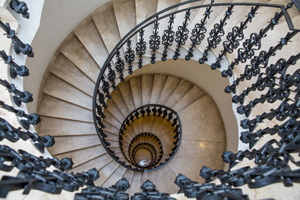Every time I come home I have to climb a flight of stairs. When I'm feeling energetic I sometimes take two steps at a time. This gives me a number of ways to climb the stairs. For example, if there are ten steps, I could climb them taking five leaps of two, giving the pattern

2, 2, 2, 2, 2.

Or I could only use a leap of two at the beginning and the end, giving the patter

2, 1, 1, 1, 1, 1, 1, 2.

How many ways are there all together of climbing the ten steps?

Being a mathematician, I don’t have ten steps of course, but I have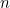steps. Can you find a formula to express the number of ways there of climbingsteps using leaps of one and two?

### Solution

Let’s write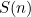for the number of ways to climb a flight of stairs withsteps. There are two possibilities for starting my climb: I could start it using a single step or a leap of two steps. If it’s the former, then there are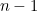steps left to climb, which I can do in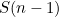ways. If it’s the latter, there are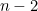steps left to climb, which I can do in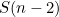ways. This means that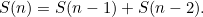This is a recurrence relation. If I know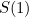and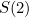then I can work out, which I can then use to work out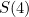, then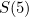, etc.

Clearly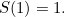There is only one way I can climb up one step and that’s using, well, one step. It’s also easy to see that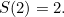I can climb up two steps using two single steps or one leap of two. This gives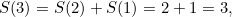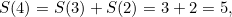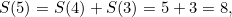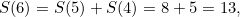and so on. This sequence of numbers might start to look familiar. It's the Fibonacci sequence, which happens to be defined via a recurrence relation in exactly the same way as we defined it here: each number is the sum of the two previous ones. (To be totally accurate, the Fibonacci sequence actually has the first two terms being equal to 1 and 1, rather than 1 and 2, so what we have here is the sequence displaced by a term.)

Carrying on with the calculations we see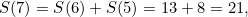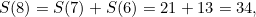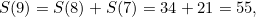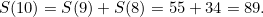So there are 89 ways of climbing a flight of ten steps using steps of one and two!